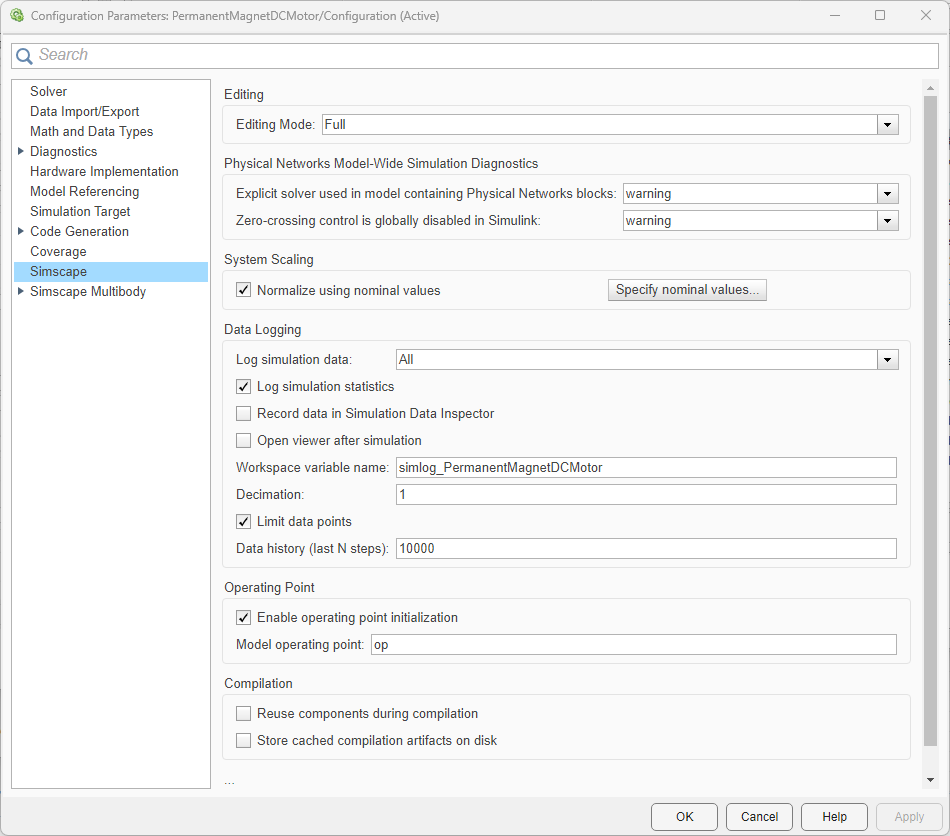## Using Operating Point Data for Model Initialization

### Using Operating Points to Initialize Model Variables

Block-level variable initialization lets you specify the priority and target for individual block variables. You can also initialize variables for a whole model from the saved operating point data.

You can use `OperatingPoint` objects to save sets of data necessary to initialize a model, manipulate this data, and then use it to initialize another model, or the same model before another simulation run. These sets of data contain a hierarchy of variable initialization targets. Each target consists of a variable value, unit, and initialization priority, as described in Variable Initialization Priority.

The `OperatingPoint` data hierarchy is a tree, with nodes corresponding to subsystems and blocks in a model. At the lowest level of the data tree, inside the block nodes, are the variable initialization targets for that block.

When you use an `OperatingPoint` to initialize a model, the solver matches the `OperatingPoint` data hierarchy to the model hierarchy and applies the initialization targets from the operating point to the respective model variables. If there is no variable matching an operating point target, this target is ignored. After applying all the data from the operating point, the solver performs model initialization as described in Initial Conditions Computation.

After you initialize the variables and prior to simulating the model, you can open the Variable Viewer to see which of the variable targets have been satisfied. For details, see Variable Viewer.

### Suggested Workflow

1. Create an `OperatingPoint` object by extracting data from the model or from the simulation log. For more information, see Extracting Variable Initialization Data into an Operating Point.

2. Modify the operating point data, if needed, by changing, adding, or removing targets and nodes. For more information, see Manipulating Operating Point Data.

3. When satisfied with the operating point data, apply it to initialize another model, or the same model for another simulation run. For more information, see Applying Operating Point Data to Initialize Model.

### Extracting Variable Initialization Data into an Operating Point

You can create an `OperatingPoint` object by extracting data from an existing model or from logged simulation data. For more information, see `simscape.op.create`.

You can extract variable initialization targets from a model in these ways:

• Start values — Initialize the model and use the variable targets corresponding to the `Start` values in the Variable Viewer.

• Prestart values — Update the model and use the variable targets corresponding to the `Prestart` values in the Variable Viewer.

• Cached data — Extract cached values of variable targets from a model that has been previously initialized or simulated. You can specify `Start` or `Prestart` values. This method lets you save time by avoiding repeated initialization of the model if the data that you want to extract has not changed.

Alternatively, you can simulate the model while logging simulation data, and then extract variable targets from the simulation log at a specified time, `t`:

• If the set of times recorded in the simulation data log contains an exact match for time `t`, then the `simscape.op.create` function extracts these variable target values into the operating point data.

• If there is no exact match, but `t` is between the minimum and maximum times in the simulation data log, then the function uses linear interpolation to determine the target values.

• If `t` is less than the minimum time, then the function extracts the first value for each variable in the simulation data log.

• If `t` is greater than the maximum time, then the function extracts the last value for each variable in the simulation data log.

When you extract data from a model into an operating point, the elements in the data hierarchy of the `OperatingPoint` object match the structure of the model. The operating point data tree has nodes corresponding to subsystems and blocks in the model, with variable initialization targets for each block at the lowest level of the data tree hierarchy. Similarly, when you extract an operating point from logged simulation data, the operating point data tree matches the data tree of the simulation log. For an example, see Find Relative Path to Block Node in Operating Point Data Tree.

### Manipulating Operating Point Data

You can create an empty `OperatingPoint` object, or populate it with the data extracted from an existing model or from logged simulation data.

Once you create an `OperatingPoint` object, you can modify it in these ways:

### Applying Operating Point Data to Initialize Model

To initialize a model from an operating point:

1. Open the Configuration Parameters dialog box.

2. On the Simscape pane, select the Enable operating point initialization check box.

3. In the Model operating point textbox, enter the name of the workspace variable associated with an `OperatingPoint` object.You can also use the equivalent command-line interface to set the model configuration parameters:

• `set_param('model_name','SimscapeUseOperatingPoints','on');`

• `set_param('model_name','SimscapeOperatingPoint','op_name');`

where `model_name` is the name of the model and `op_name` is the name of the `OperatingPoint` object.